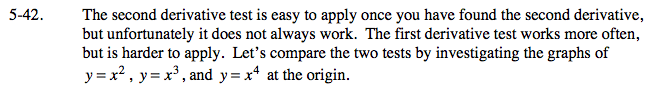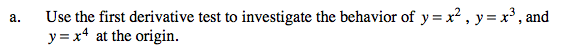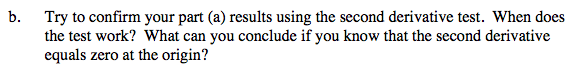### Home > CALC > Chapter 5 > Lesson 5.1.4 > Problem5-42

5-42.When f '(a) = 0, f(x) could be at a local max, a local min, or neither (it might be a point of inflection).
The 1st and 2nd derivative tests are ways to tell what is going on at x = a.Directions: Evaluate f '(x) at two points, one to the left of x = a and the other to the right of x = a.
☛ If the sign changes from negative to positive, then f(x) changes from decreasing to increasing and f(a) is a local min.
☛ If the sign changes from positive to negative, then f(x) changes from increasing to decreasing and f(a) is a local max.
☛ If the sign does not change, then f(x) is either increasing or decreasing (depending on the sign).Directions: Evaluate f "(a)
☛ If f "(a) > 0, then f(x) is concave up at x = a, and f(a) is a local min.
☛ If f "(a) < 0, then f(x) is concave down at x = a, and f(a) is a local max.
☛ If f "(a) = 0, then this test is inconclusive.